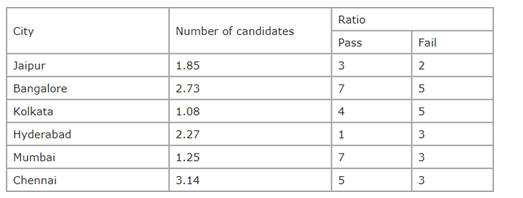# “20-20” Quantitative Aptitude | Crack SBI Clerk 2018 Day-3

Dear Friends, SBI Clerk 2018 Notification has been released we hope you all have started your preparation. Here we have started New Series of Practice Materials specially for SBI Clerk 2018. Aspirants those who are preparing for the exams can use this “20-20” Quantitative Aptitude Questions.

[WpProQuiz 1132]

### Click Here for SBI Clerk Prelims and Mains 2018 – Full Length Mock Test

1. Perimeter of a rectangle is x and circumference of a circle is 8 more than the perimeter of the rectangle. Ratio of radius of circle and length of the rectangle is 1 : 2 and ratio of length and breadth of rectangle is 7 : 3. Find the length of the rectangle?
1. 14
2. 21
3. 28
4. 35
5. 7
1. A, B and C started a business with their investments in the ratio 1 : 2 : 4. After 6 month A invested the half amount more as before and B invested same the amount as before while C withdrew 1/4th of his investment. Find the ratio of their profits at the end of the year.
1. 5 : 12 : 13
2. 5 : 11 :14
3. 5: 12 : 14
4. 5 : 12 : 10
5. None of the above
1. The average profit earned by a trader in the first three days of a week is Rs.6750. The average loss for the next two days is Rs.3850. What should he the average loss/ gain per day for the last two days so that there is no loss or gain in the whole week?
1. Rs.6725
2. Rs.6275
3. Rs.6325
4. Rs.6250
5. Other than the given options
1. A sum of money lent at compound interest at the rate of 10% per annum is paid back in three equal annual installments of Rs.2662. Find the sum of money.
1. Rs.6200
2. Rs.6260
3. Rs.6600
4. Rs.6620
5. Other than the given options
1. Harish brother is 3 years elder than Harish. Harish father was 28 years of age when Harish sister was born while Harish mother was 26 years of age when Harish was born. If Harish sister was 4 years of age when Harish brother was born, then what was the age Harish father when Harish brother was born?
1. 36 years
2. 30 years
3. 31 years
4. 32 years
5. None of these

Directions (Q. 6-10): Study the tables carefully and answer the questions that follow: Number of candidates (in lakhs) appearing in an entrance examination from six different cities and the ratio of candidates passing and failing in the same1. What is the difference between the city has the highest number of passing students in the exam to the city has the lowest number of students failing in the exam?
1. 132750
2. 168750
3. 164750
4. 158750
5. None of these
1. Number of candidates passing in the exam from Bangalore is what percent of the total number of candidates appearing from all the Cities together? (rounded off to two digits after the decimal)
1. 12.93
2. 11.46
3. 13.84
4. 15.27
5. None of these
1. The number of candidates passed from Jaipur and Kolkata together is what percent of the total number of failed candidates from Hyderabad?
1. 95.78%
2. 91.28%
3. 95.49%
4. 92.01%
5. 93.39%
1. What is the respective ratio of the number of candidates failing in the Exam from Hyderabad and Jaipur together to those failing in the exam from Mumbai and Chennai together?
1. 927 : 623
2. 621 : 977
3. 977 : 621
4. 947 : 459
5. None of these
1. The number of candidates appearing for the exam from Kolkata and Mumbai together is what percent of the total number of candidates appearing for the exam from Chennai and Bangalore together? (rounded off to the nearest integer)
1. 45
2. 40
3. 35
4. 33
5. 30

Directions (Q.11-15): In each, these questions a number series is given. In each series only one number is missing. Find out the number.

11). 97, 108, 130, 163, ?,   262

1. 230
2. 210
3. 217
4. 207
5. 202

12). 15,  28,  45,  66 , ? ,  120

1. 105
2. 86
3. 98
4. 91
5. 84

13). 65,  83,  105,  131,  161, ?

1. 140
2. 165
3. 195
4. 194
5. 160

14). 11,  25,  55,  117,   ?,   497

1. 243
2. 235
3. 248
4. 234
5. 245

15). 1,  4,   19,  54,  117,   ?

1. 195
2. 216
3. 215
4. 205
5. 208

Directions (Q. 16-20) : What approximate value should come in place of question mark (?) in the given number series ?

16). 17.98^2 + 4.05 x 90.11 ÷ 4.98 = ?

1. 396
2. 336
3. 242
4. 423
5. 816

17). 80.04% of 150.16 + 60.02% of 50.07 = ?

1. 150
2. 125
3. 210
4. 175
5. 213

18). √628 × 17.996 ÷ 15.04 = ?

1. 30
2. 10
3. 5
4. 20
5. 25

19). 29/143 of 14.3% of 37/300 of ? = 28

1. 7840
2. 8480
3. 1020
4. 1260
5. None of these

20). (20.89% of 425.95 + 17.67% of 135.986) = √?

1. 9409
2. 10609
3. 12769
4. 13689
5. None of these